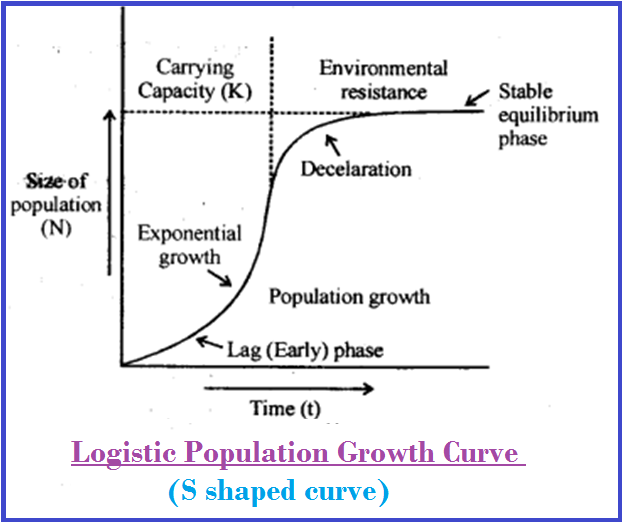Biology GROWTH MODELS

### KEY TOPICS

star Exponential Growth
star Logistic Growth

### EXPONENTIAL GROWTH● Resource (color{violet}("food and space")) availability is obviously essential for the color{violet}("unimpeded growth of a population").

● Ideally, when resources in the habitat are unlimited, each species has the ability to realise fully its color{violet}("innate potential to grow") in number, as color{violet}("Darwin observed") while developing his color{violet}("theory of natural selection.")

● Then the color{violet}("population grows") in an exponential or color{brown}("geometric fashion.")

● If in a color{violet}("population of size") N, the color{violet}("birth rates (not total number but per capita births)") are represented as b and color{violet}("death rates (again, per capita death rates)") as d,

then the increase or decrease in N during a color{violet}("unit time period") color{violet}("t (dN/dt)") will be

color{violet}("dN/dt = (b – d) × N")

Let color{violet}((b–d) = r), then

color{violet}("dN/dt = rN")

● The color{brown}(r) in this equation is called thecolor{brown}("intrinsic rate") of color{brown}("natural increase") and is a very important parameter chosen for assessing impacts of any color{violet}("biotic or abiotic factor") on color{violet}("population growth.")

● To give you some idea about the color{violet}("magnitude") of color{violet}(r) values, for the color{violet}("Norway rat") the color{violet}(r) is 0.015, and for the color{violet}("flour beetle") it is 0.12.

● color{violet}("In 1981"), the color{violet}(r) value for color{violet}("human population") in India was 0.0205.

● The above equation describes the color{brown}("exponential or geometric growth pattern") of a population and results in a color{brown}("J-shaped curve") when we plot N in relation to time.

● If you are familiar with basic calculus, you can derive the integral form of the color{violet}("exponential growth equation") as

color{violet}(N_t = N_0 e^(rt))
where
color{violet}(N_t) = Population density after time color{violet}(t)
color{violet}(N_0) = Population density at time zero
color{violet}(r) = intrinsic rate of natural increase
color{violet}(e) = the base of natural logarithms color{violet}((2.71828))

● Any species color{violet}("growing exponentially") under unlimited resource color{violet}("conditions") can reach color{violet}("enormous population") color{violet}("densities") in a short time.

● color{violet}("Darwin showed") how even a color{violet}("slow growing animal") like elephant could reach color{violet}("enormous numbers") in the color{violet}("absence of checks").

### LOGISTIC GROWTH● No population of any species in nature has its color{violet}("disposal unlimited resources") to permit color{violet}("exponential growth").

● This leads to competition between individuals for color{violet}("limited resources.")

● Eventually, the color{violet}("‘fittest’") individual will color{violet}("survive") and color{violet}("reproduce").

● The governments of many countries have also realised this fact and introduced various color{violet}("restraints") with a view to color{violet}("limit human population growth.")

● color{violet}("In nature,") a given habitat has enough resources to support a color{violet}("maximum possible number"), beyond which no further color{violet}("growth is possible.")

● This limit is called as color{brown}("nature’s carrying capacity (K)") for that species in that habitat.

● A color{violet}("population growing") in a habitat with limited resources show color{violet}("initially a lag phase,") followed by phases of color{violet}("acceleration and deceleration") and finally an color{violet}("asymptote,") when thecolor{violet}(" population density") reaches the color{violet}("carrying capacity").

● A plot of N in relation to time (t) results in acolor{brown}("sigmoid curve").

● This type of color{violet}("population growth") is called color{brown}("Verhulst-Pearl Logistic Growth") and is described by
the color{violet}("following equation"):

color{violet}(dN/dt = rN ((K-N)/K)
Where color{violet}(N) = Population density at time color{violet}(t)
color{violet}(r) = Intrinsic rate of natural increase
color{violet}(K) = Carrying capacity

● Since resources for color{violet}("growth") for most color{violet}("animal populations") are color{violet}("finite") and become limiting sooner or later, the color{violet}("logistic growth model") is considered a more realistic one.